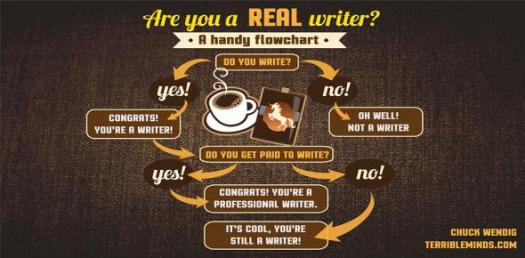# Basic Symbols Of Flowchart! Trivia Quiz

10 Questions | Total Attempts: 463SettingsA Flowchart is a diagrammatic description of a process. The flowchart show how a process is carried out step by step and employs the use of basic symbols. What do you know about Flowchart? What do you understand about the basic symbols of a flowchart? Take our quiz to discover everything you know on it

• 1.
What is used to represent an end?
• A.

Oval

• B.

Rectangle

• C.

Arrow

• D.

Diamond

• 2.
What is used in representing the beginning of a process?
• A.

Oval

• B.

Rectangle

• C.

Arrow

• D.

Diamond

• 3.
Which of these is a 4-angled flowcharting process?
• A.

Oval

• B.

Rectangle

• C.

Arrow

• D.

Diamond

• 4.
What symbol is used to give directions?
• A.

Oval

• B.

Rectangle

• C.

Arrow

• D.

Diamond

• 5.
What is a call to decision symbol?
• A.

Oval

• B.

Rectangle

• C.

Arrow

• D.

Diamond

• 6.
What represent input or output?
• A.

Parallelogram

• B.

Rectangle

• C.

Line

• D.

Diamond

• 7.
What shows relationship?
• A.

Parallelogram

• B.

Rectangle

• C.

Line

• D.

Diamond

• 8.
What indicates decision?
• A.

Parallelogram

• B.

Rectangle

• C.

Line

• D.

Diamond

• 9.
What represents a process?
• A.

Parallelogram

• B.

Rectangle

• C.

Line

• D.

Diamond

• 10.
What is a connector symbol?
• A.

Circle

• B.

Hexagon

• C.

Triangle

• D.

Arrow

Related TopicsBack to top